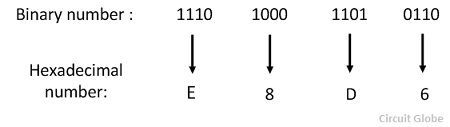The hexadecimal number are mostly used in microprocessor work. The base of the hexadecimal number is 16 and its consist the number from 0 -15. It uses the number system from 0-9 and then represents by the alphabets from A- F. The hexadecimal number system is also called hex. It is one of the convenient ways of describing the  binary digit number. The large binary digits are easily represented by the hexadecimal number.

The base of the binary number system is two because it is represented by two digits, i.e., 0 and 1. It is difficult to represent the large number in the form of binary digit and hence the hexadecimal system are used in the digital electronics.The hexadecimal number systems are easily converted into a binary system by using the method explains below.

###Hexadecimal to Binary Conversion Method

To convert a hexadecimal number to a binary number, convert each hexadecimal digit to its four digit equivalent. For example, consider the hexadecimal number 9AF which is converted into a binary digit. The conversions are explained below.Therefore the equivalent binary number is 1001 1010 1111.

### Binary to Hexadecimal Conversion Methods

To convert the given binary number into its equivalent hexadecimal number rewrite the binary number of the sets of four digits and then place the hexadecimal digit in front of each four digit set of a binary number as explained by the following number.Thus the equivalent hexadecimal number is E8D6.